# C109 – Pulse Output Meter Calibration

## Description

This calculation can be set to solve the K-factor, meter factor, corrected prover volume and the required pulses.

Institute of Petroleum – Petroleum Measurement Manual: Part X – Meter Proving

Kelton calculation reference C109

KIMS calculation reference K136

## Options

### Calculate

• Meter K-factor
• Meter factor
• Corrected volume
• Required pulses

This option group is used to select the value(s) for the calculation to solve.

The remaining option logic is the same as for C108 when “Pipe prover” is selected.

## Calculation

### Ctsp

The Ctsp is calculated by: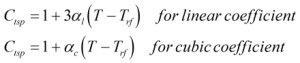Where αc = Cubic temperature coefficient αl = Linear temperature coefficient Tp = Prover temperature Trf = Prover reference temperature

### Cpsp

The Cpsp is calculated by: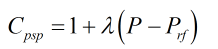Where λ = Pressure coefficient Pp = Prover pressure Prf = Prover reference pressure

### K-factor

The K-factor is calculated by: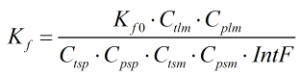Where Kf0 = Uncorrected K-factor Ctlm = Correction for temperature of the liquid at the meter Cplm = Correction for pressure of the liquid at the meter Ctlp = Correction for temperature of the liquid at the prover Cplp = Correction for pressure of the liquid at the prover Ctsp = Correction for temperature on steel of the prover Cpsp = Correction for pressure on steel of the prover IntF = Interpolation factor

### Meter Factor

The meter factor is calculated by: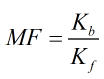Where Kb = Base K-factor Kf = K-factor

### Corrected volume

The corrected prover volume is calculated by: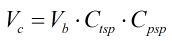Where Vb = Base prover volume

### Required pulses

The number of required pulses is calculated by:Where nm = Number of pulses

Back